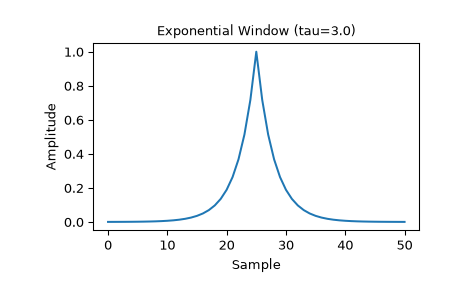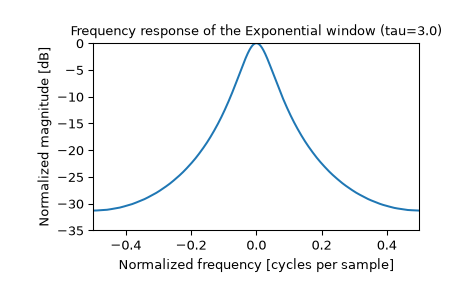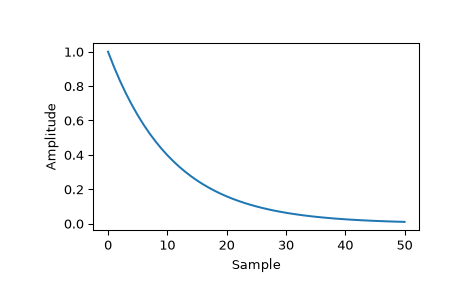# scipy.signal.windows.exponential¶

scipy.signal.windows.exponential(M, center=None, tau=1.0, sym=True)[source]

Return an exponential (or Poisson) window.

Parameters
Mint

Number of points in the output window. If zero or less, an empty array is returned.

centerfloat, optional

Parameter defining the center location of the window function. The default value if not given is center = (M-1) / 2. This parameter must take its default value for symmetric windows.

taufloat, optional

Parameter defining the decay. For center = 0 use tau = -(M-1) / ln(x) if x is the fraction of the window remaining at the end.

symbool, optional

When True (default), generates a symmetric window, for use in filter design. When False, generates a periodic window, for use in spectral analysis.

Returns
wndarray

The window, with the maximum value normalized to 1 (though the value 1 does not appear if M is even and sym is True).

Notes

The Exponential window is defined as

$w(n) = e^{-|n-center| / \tau}$

References

S. Gade and H. Herlufsen, “Windows to FFT analysis (Part I)”, Technical Review 3, Bruel & Kjaer, 1987.

Examples

Plot the symmetric window and its frequency response:

>>> from scipy import signal
>>> from scipy.fft import fft, fftshift
>>> import matplotlib.pyplot as plt

>>> M = 51
>>> tau = 3.0
>>> window = signal.exponential(M, tau=tau)
>>> plt.plot(window)
>>> plt.title("Exponential Window (tau=3.0)")
>>> plt.ylabel("Amplitude")
>>> plt.xlabel("Sample")

>>> plt.figure()
>>> A = fft(window, 2048) / (len(window)/2.0)
>>> freq = np.linspace(-0.5, 0.5, len(A))
>>> response = 20 * np.log10(np.abs(fftshift(A / abs(A).max())))
>>> plt.plot(freq, response)
>>> plt.axis([-0.5, 0.5, -35, 0])
>>> plt.title("Frequency response of the Exponential window (tau=3.0)")
>>> plt.ylabel("Normalized magnitude [dB]")
>>> plt.xlabel("Normalized frequency [cycles per sample]")


This function can also generate non-symmetric windows:

>>> tau2 = -(M-1) / np.log(0.01)
>>> window2 = signal.exponential(M, 0, tau2, False)
>>> plt.figure()
>>> plt.plot(window2)
>>> plt.ylabel("Amplitude")
>>> plt.xlabel("Sample")